## Imagine a unit of charge called the zorg. A chemist performsthe oil drop experiment and measures the charge of each drop inzorgs. Based on t

Question

Imagine a unit of charge called the zorg. A chemist performsthe oil drop experiment and measures the charge of each drop inzorgs. Based on the results below, what is the charge of theelectron in zorgs (z)? How many electrons are in eachdrop?
Drop # Charge
A -4.8 x 10^-9 z
B -9.6 x 10^-9 z
C -6.4 x 10^-9 z
D -12.8 x 10^9z

in progress 0
2 months 2021-07-25T16:44:21+00:00 1 Answers 2 views 0

-1.6 × 10⁻⁹ z

Explanation:

To attempt this type of question, we need to first divide each charge present in the question with the smallest one.

i.e.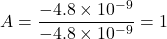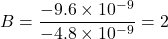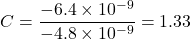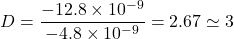The next thing to do is to multiply each obtained value with the highest integer

A = 1 × 3 = 3

B = 2 × 3 = 6

C = 1.33 × 3 = 3.99

D = 3 × 3 = 9

Finally, we divide each charge by the result from above.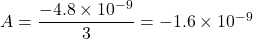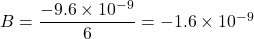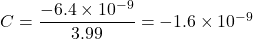Thus, we can therefore easily conclude that the charge in zorgs (z) is:

-1.6 × 10⁻⁹ z